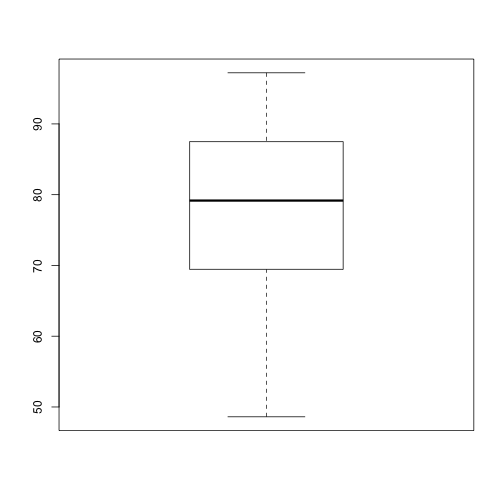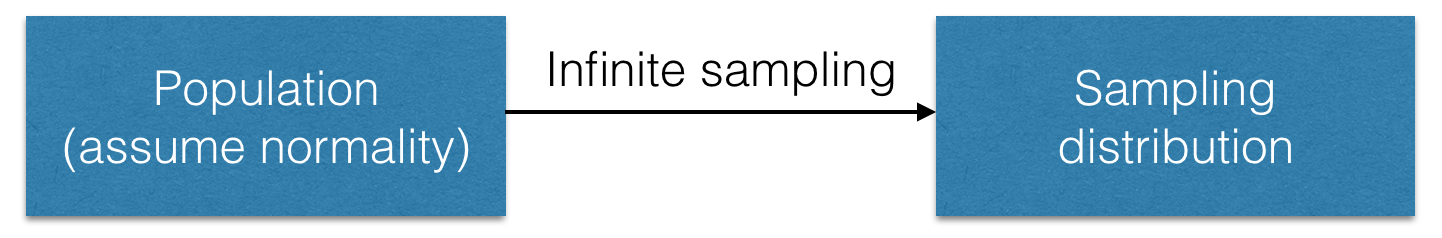The analysis of variance

M. Drew LaMar
April 6, 2016

Class announcements

• Exam #3 is on Friday, April 22.

summary(grades$Exam2) Min. 1st Qu. Median Mean 3rd Qu. Max. NA's 48.61 69.44 79.17 78.12 87.50 97.22 1 Exam #2 boxplot(grades$Exam2, datax=TRUE)Permutation tests

Definition: A permutation test generates a null distribution for the association between two variables by repeatedly and randomly rearranging the values of one of the two variables in the data.

This is a form of bootstrapping.Permutation tests for association

In this chapter, we explore a permutation test replacement for the two-sample $$t$$-test.

Variations on this theme can be done for many other tests.

Permutation tests for association

ParametricPermutationPermutation tests

Algorithm

• Choose a test statistic that measures association between the two variables in the data (e.g. $$\bar{Y}_{1}-\bar{Y}_{2}$$; Why not $$t$$?)
• Create a permuted sample of data in which the values of the response variables are randomly reordered.
• Calculate the chosen test statistic for the permuted sample.
• Repeat the permutation process many times – at least 1000 or more.
• Compute the $$P$$-value by comparing the observed test statistic (from original data) to this bootstrapped null distribution.